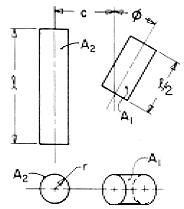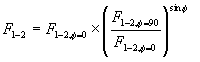# SECTION C Factors From Finite Areas to Finite Areas

## C-107: Cylinder of length l/2 to cylinder of equal radius and length l rotated in plane containing cylinder axes about a line through end of longer cylinder.Definitions:
 L = l/r;

Governing Equation:Where F1-2,f=0 is the value computed for equal length and radius parallel directly opposed cylinders of length l (configuration C-101), and F1-2,f=90 is from the preceding entry in this catalog (C-106).
To download a copy of this equation in MS Word 97 Format, click HERE.